# Logarithmic function, graph of logarithmic function

Definition: the Logarithmic function is a function of the form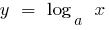where### Properties of logarithmic functions

1. Scope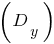2.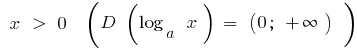3. Multiple value4.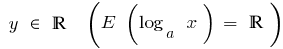5. Parity, odd parity
6. The function is neither even nor odd

7. The intersection with the coordinate axes
8.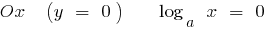when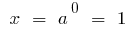Of intersection with the axis of the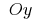no(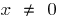scope)

9. Continuity and derivative
10. The function is continuous and diferencian in the whole scope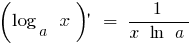11. The intervals of constancy of sign
12.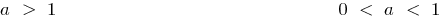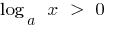when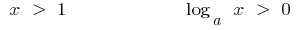in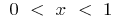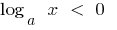when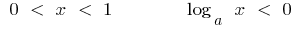in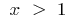13. The increase and decrease of
14. Extrema no

in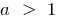— growing

when itcomes

15. Asymptotes
16. Video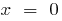— vertical asymptote

Functions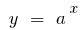andmutually inverse functions, so the Graphs are symmetric with respect to the line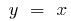### Graphs of logarithmic functions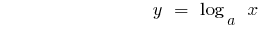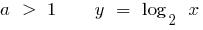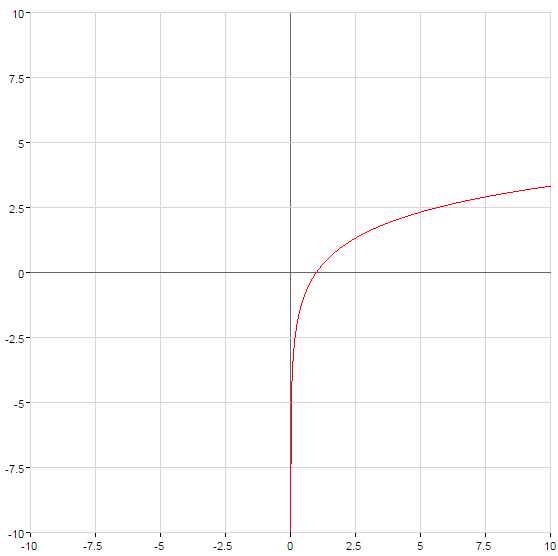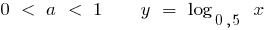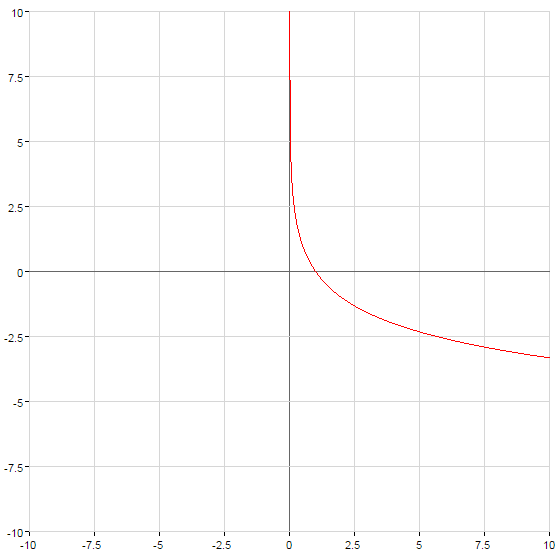Tags:
Chapter:
Versions in other languages:
Share with friends: